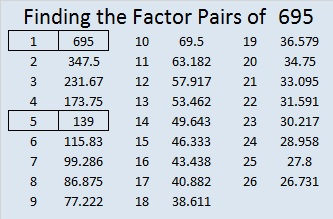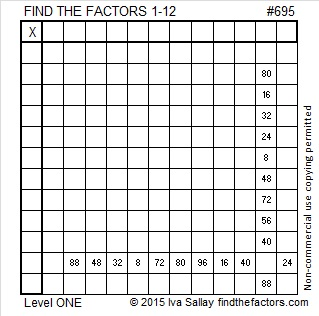# 695 and Level 1

• 695 is a composite number.
• Prime factorization: 695 = 5 x 139
• The exponents in the prime factorization are 1 and 1. Adding one to each and multiplying we get (1 + 1)(1 + 1) = 2 x 2 = 4. Therefore 695 has exactly 4 factors.
• Factors of 695: 1, 5, 139, 695
• Factor pairs: 695 = 1 x 695 or 5 x 139
• 695 has no square factors that allow its square root to be simplified. √695 ≈ 26.36285.Here is today’s puzzle:Print the puzzles or type the solution on this excel file: 12 Factors 2015-11-30

———————————————————————————

Here are a few more facts about the number 695:

695 is the sum of the eighteen prime numbers from 7 to 73.

695 is also the hypotenuse of the Pythagorean triple 417-556-695. What is the greatest common factor of those three numbers?

137 + 138 + 139 + 140 + 141 = 695 so it is the sum of 5 consecutive numbers.

Since 695 is odd, it is the sum of two consecutive numbers, 347 and 348.

———————————————————————————